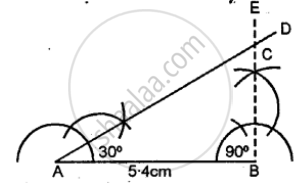# Construct Traingle Abc, When : Ab = 5.4 Cm, ∠A = 30° and ∠B = 90°. Measure ∠C and Side Bc. - Mathematics

Sum

Construct traingle ABC, when : AB = 5.4 cm, ∠A = 30° and ∠B = 90°. Measure ∠C and side BC.

#### Solution

Steps of Construction:

(1) Draw a line segment AB = 5.4 cm.(2) At A construct an angle ∠A = 30°.

(3) At B construct an angle ∠B = 90°.

(4) AD and BE intersect each other at C.

(5) ∴ ∆ABC is the required triangle.

(6) On measuring ∠C = 60° side BC = 31 cm.

Concept: Classification of Triangles (On the Basis of Sides, and of Angles)
Is there an error in this question or solution?

#### APPEARS IN

Selina Class 6 Mathematics
Chapter 26 Triangles (Including Types, Properties and Constructions)
Exercise 26 (B) | Q 8